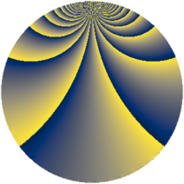# Properties

 Label 775.2.fLevel $775$ Weight $2$ Character orbit 775.f Rep. character $\chi_{775}(557,\cdot)$ Character field $\Q(\zeta_{4})$ Dimension $92$ Newform subspaces $7$ Sturm bound $160$ Trace bound $31$

# Related objects

## Defining parameters

 Level: $$N$$ $$=$$ $$775 = 5^{2} \cdot 31$$ Weight: $$k$$ $$=$$ $$2$$ Character orbit: $$[\chi]$$ $$=$$ 775.f (of order $$4$$ and degree $$2$$) Character conductor: $$\operatorname{cond}(\chi)$$ $$=$$ $$155$$ Character field: $$\Q(i)$$ Newform subspaces: $$7$$ Sturm bound: $$160$$ Trace bound: $$31$$ Distinguishing $$T_p$$: $$2$$, $$3$$

## Dimensions

The following table gives the dimensions of various subspaces of $$M_{2}(775, [\chi])$$.

Total New Old
Modular forms 172 100 72
Cusp forms 148 92 56
Eisenstein series 24 8 16

## Trace form

 $$92 q + 4 q^{2} + 12 q^{7} - 10 q^{8} + O(q^{10})$$ $$92 q + 4 q^{2} + 12 q^{7} - 10 q^{8} - 40 q^{16} - 16 q^{18} - 38 q^{28} + 4 q^{32} + 44 q^{33} + 16 q^{36} + 38 q^{38} + 24 q^{41} - 24 q^{47} + 40 q^{51} + 104 q^{56} - 44 q^{62} - 40 q^{63} - 104 q^{66} + 12 q^{67} - 48 q^{71} - 98 q^{72} - 24 q^{76} - 104 q^{78} - 28 q^{81} - 34 q^{82} + 20 q^{87} + 60 q^{93} + 72 q^{97} - 46 q^{98} + O(q^{100})$$

## Decomposition of $$S_{2}^{\mathrm{new}}(775, [\chi])$$ into newform subspaces

Label Dim $A$ Field CM Traces $q$-expansion
$a_{2}$ $a_{3}$ $a_{5}$ $a_{7}$
775.2.f.a $4$ $6.188$ $$\Q(i, \sqrt{6})$$ None $$0$$ $$-4$$ $$0$$ $$0$$ $$q+\beta _{1}q^{2}+(-1-\beta _{2})q^{3}+\beta _{2}q^{4}+(-\beta _{1}+\cdots)q^{6}+\cdots$$
775.2.f.b $4$ $6.188$ $$\Q(i, \sqrt{6})$$ None $$0$$ $$4$$ $$0$$ $$0$$ $$q+\beta _{1}q^{2}+(1+\beta _{2})q^{3}+\beta _{2}q^{4}+(\beta _{1}+\cdots)q^{6}+\cdots$$
775.2.f.c $8$ $6.188$ 8.0.3317760000.2 None $$0$$ $$0$$ $$0$$ $$0$$ $$q-\beta _{6}q^{2}-\beta _{3}q^{3}-4\beta _{2}q^{4}-\beta _{7}q^{6}+\cdots$$
775.2.f.d $8$ $6.188$ 8.0.$$\cdots$$.2 $$\Q(\sqrt{-155})$$ $$0$$ $$0$$ $$0$$ $$0$$ $$q+\beta _{3}q^{3}+2\beta _{4}q^{4}+(-3\beta _{4}-\beta _{7})q^{9}+\cdots$$
775.2.f.e $12$ $6.188$ 12.0.$$\cdots$$.1 $$\Q(\sqrt{-31})$$ $$0$$ $$0$$ $$0$$ $$0$$ $$q-\beta _{4}q^{2}+(2\beta _{1}-\beta _{2})q^{4}+(-\beta _{2}+\beta _{9}+\cdots)q^{7}+\cdots$$
775.2.f.f $16$ $6.188$ $$\mathbb{Q}[x]/(x^{16} - \cdots)$$ None $$4$$ $$0$$ $$0$$ $$12$$ $$q+\beta _{1}q^{2}-\beta _{4}q^{3}+(\beta _{1}+\beta _{2})q^{4}+(\beta _{7}+\cdots)q^{6}+\cdots$$
775.2.f.g $40$ $6.188$ None $$0$$ $$0$$ $$0$$ $$0$$

## Decomposition of $$S_{2}^{\mathrm{old}}(775, [\chi])$$ into lower level spaces

$$S_{2}^{\mathrm{old}}(775, [\chi]) \cong$$ $$S_{2}^{\mathrm{new}}(155, [\chi])$$$$^{\oplus 2}$$